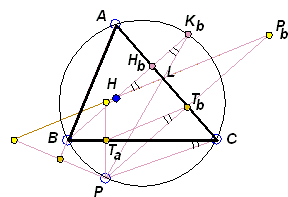# Reflections of a Point on the CircumcircleWhat Is This About? A Mathematical Droodle

### This applet requires Sun's Java VM 2 which your browser may perceive as a popup. Which it is not. If you want to see the applet work, visit Sun's website at https://www.java.com/en/download/index.jsp, download and install Java VM and enjoy the applet.

 What if applet does not run?

ExplanationCopyright © 1996-2018 Alexander Bogomolny

### Reflections of a Point on the Circumcircle

The applet suggests the following theorem [Coxeter & Greitzer, p. 45, Honsberger, pp. 43-44]:

The reflections of a point on the circumcircle of a triangle in the side lines of the latter are collinear with the orthocenter of the triangle.

Let P be a point on the circumcenter of ΔABC. Ta (Tb, Tc) is the foot of the perpendicular from P to BC and Pa (Pb, Pc) is the reflection of P in BC (AC, AB). We wish to prove that the four points Pa, Pb, Pc, and H are collinear.

Note that the points Ta, Tb, Tc lie on the simson of P with respect to ΔABC. Also, the points Pa, Pb, Pc are the images of points Ta, Tb, Tc under the homothety with center P and coefficient 2. Thus we immediately see that the points Pa, Pb, Pc are collinear. It remains to be shown that the orthocenter H lies on the same straight line.In the diagram, TaTb is the simson line ΔABC. To prove the assertion, we shall show that HPb||TaTb.

First, since PTa is perpendicular to BC and PTb is perpendicular to AC, the quadrilateral PTaTbC is cyclic. The inscribed angles TaTbP and TaCP stand on the same arc TaP and are, therefore equal:

 (1) ∠TaTbP = ∠TaCP.

HPb is the reflection of KbP in AC, while HKb is perpendicular to AC. Therefore,

 (2) ∠BKbP = ∠KbHPb.

Angles BKbP and BCP (= TaCP) are equal as inscribed into the same circle and being subtended by the same arc BP:

 (3) ∠BKbP = ∠BCP.

The combination of (1)-(3) gives a crucial for the proof identity:

 (4) ∠TaTbP = ∠PbHHb.

Since one pair of the sides of these angles (PTb and HHb are both perpendicular to AC) are parallel, the same holds for the other pair of the sides: HPb||TaTb.

### Corollary

The simson line of a point P on the circumcircle of a triangle bisects the segment PH joining the point to the orthocenter H.

### References

1. H.S.M. Coxeter, S.L. Greitzer, Geometry Revisited, MAA, 1967
2. R. Honsberger, Episodes in Nineteenth and Twentieth Century Euclidean Geometry, MAA, 1995.### Simson Line - the simson

• Simson Line: Introduction
• Simson Line
• Three Concurrent Circles
• 9-point Circle as a locus of concurrency
• Miquel's Point
• Circumcircle of Three Parabola Tangents
• Angle Bisector in Parallelogram
• Simsons and 9-Point Circles in Cyclic Quadrilateral
• Simsons of Diametrically Opposite Points
• Simson Line From Isogonal Perspective
• Pentagon in a Semicircle
• Simson Line in Disguise
• Two Simsons in a Triangle
• Carnot's Theorem
• A Generalization of Simson LineCopyright © 1996-2018 Alexander Bogomolny

 64856169